# Block A supports a pipe column and rests as shown on wedge B.Knowing that the coefficient of static friction at all surfaces of contact is 0.25 and that θ = 45°, determine the smallest force P required to raise block A.

Question-AnswerCategory: Engineering MechanicsBlock A supports a pipe column and rests as shown on wedge B.Knowing that the coefficient of static friction at all surfaces of contact is 0.25 and that θ = 45°, determine the smallest force P required to raise block A.

Block A supports a pipe column and rests as shown on wedge B.Knowing that the coefficient of static friction at all surfaces of contact is 0.25 and that θ = 45°, determine the smallest force P required to raise block A.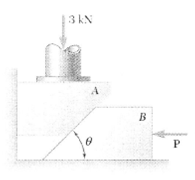Step: 1

Calculate the friction angle by using the following relation:Substitute 0.25 for.Step: 2

Draw the free body diagram of the block A.Here,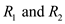are the reaction forces on block A.

Step: 3

Draw the force triangle for block A.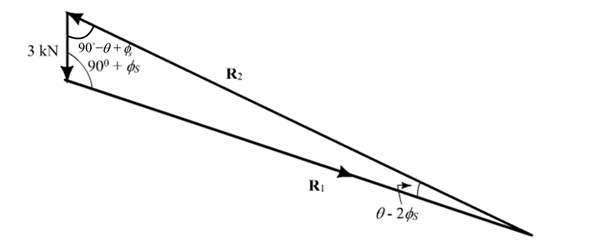Step: 4

Apply the law of sine and solve.Substitute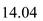for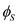and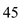for.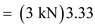Step: 5

Draw the free body diagram of wedge B.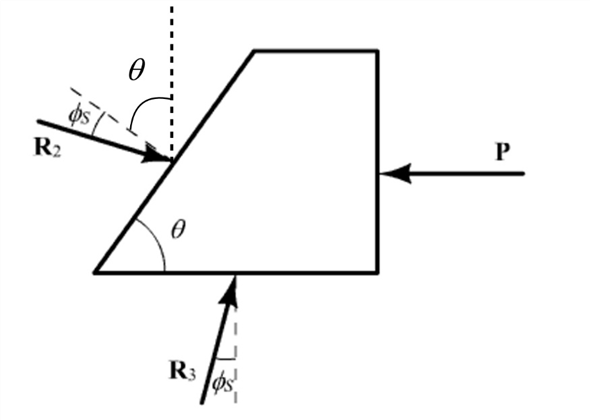Step: 6

Draw the force triangle for block B.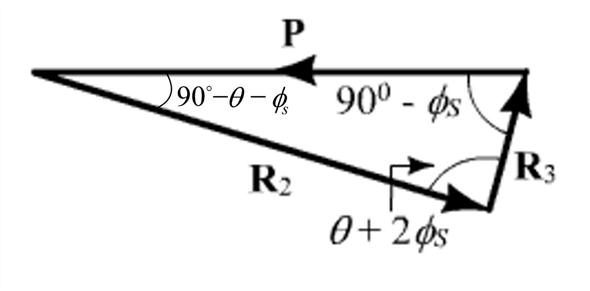Step: 7

Calculate the smallest force P.
Apply the law of sines and solve.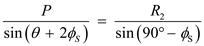Substituteforandfor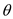.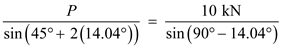Therefore, the smallest force required to raise block A is.